Algebra 2 5-9 Transforming Polynomial Functions
starstarstarstarstarstarstarstarstarstar
4 (1 rating)
by Matthew Richardson
| 23 Questions
Note from the author:
A complete formative lesson with embedded slideshow, mini lecture screencasts, checks for understanding, practice items, mixed review, and reflection. I create these assignments to supplement each lesson of Pearson's Common Core Edition Algebra 1, Algebra 2, and Geometry courses. See also mathquest.net and twitter.com/mathquestEDU.
The outlined content above was added from outside of Formative.
1
10 pts
Solve It! The graph of the parent cubic function f(x) = is shown in blue. The red graph is a transformation g of the parent function f. What is an equation for g?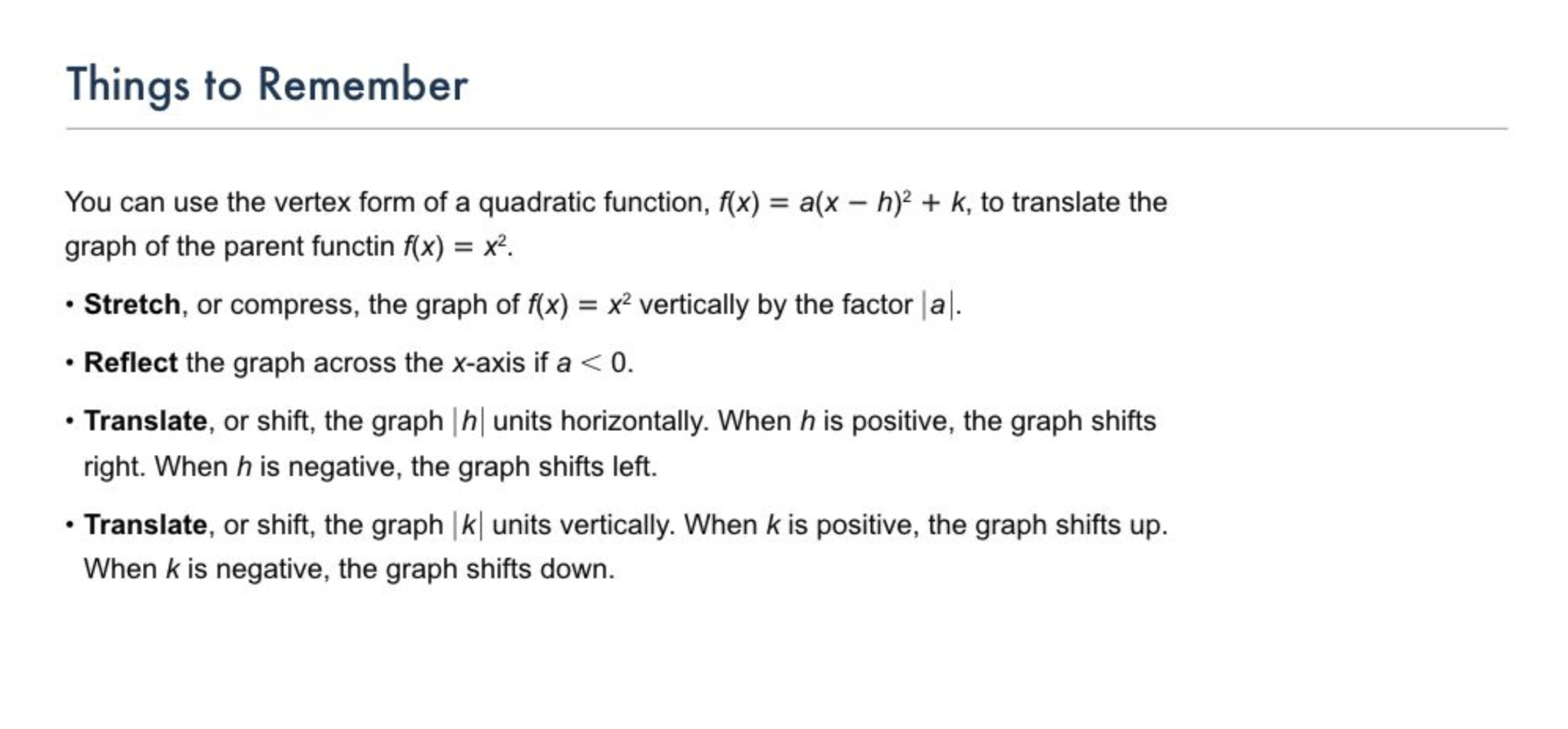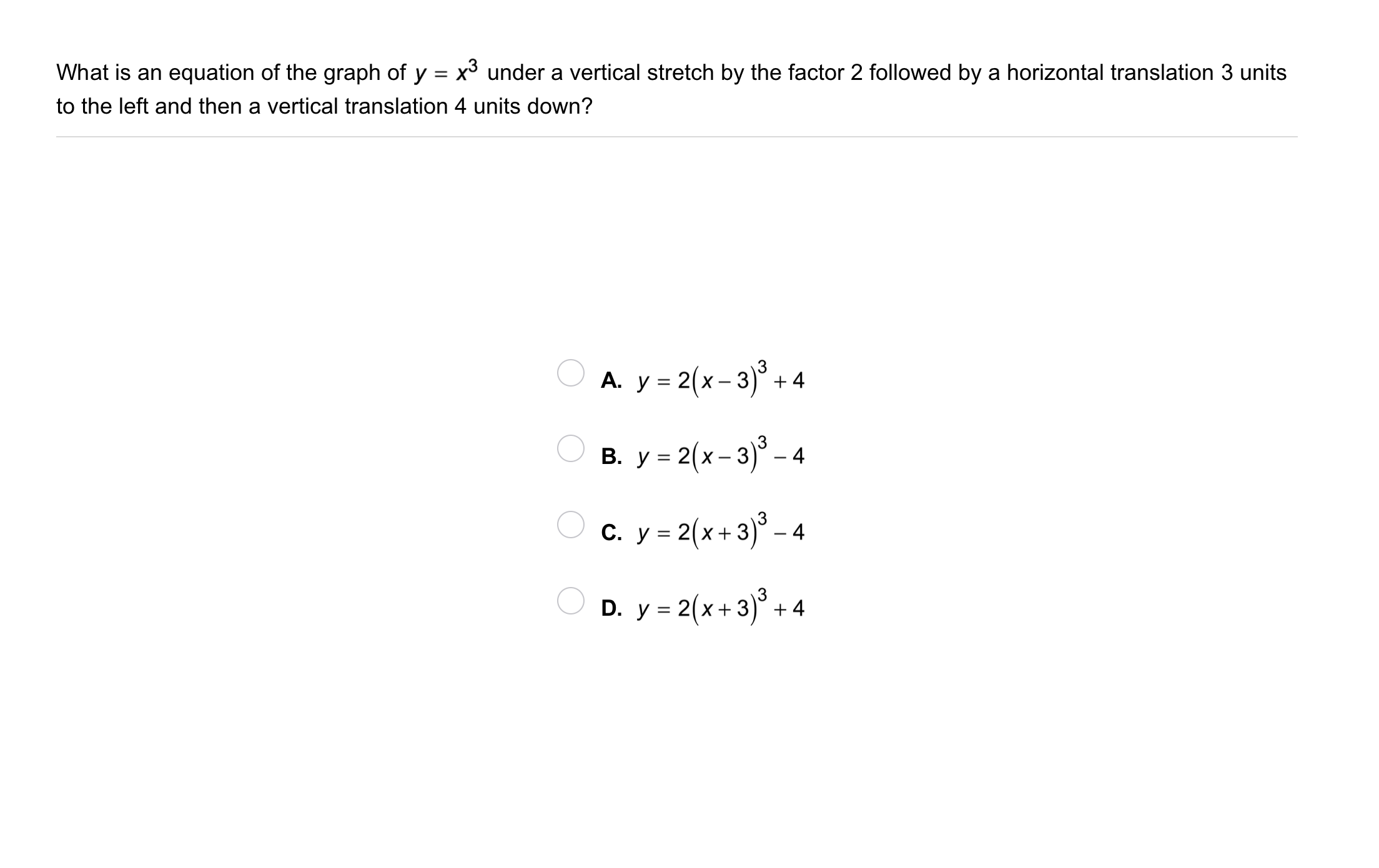2
2
10 pts
Problem 1 Got It?
A
B
C
D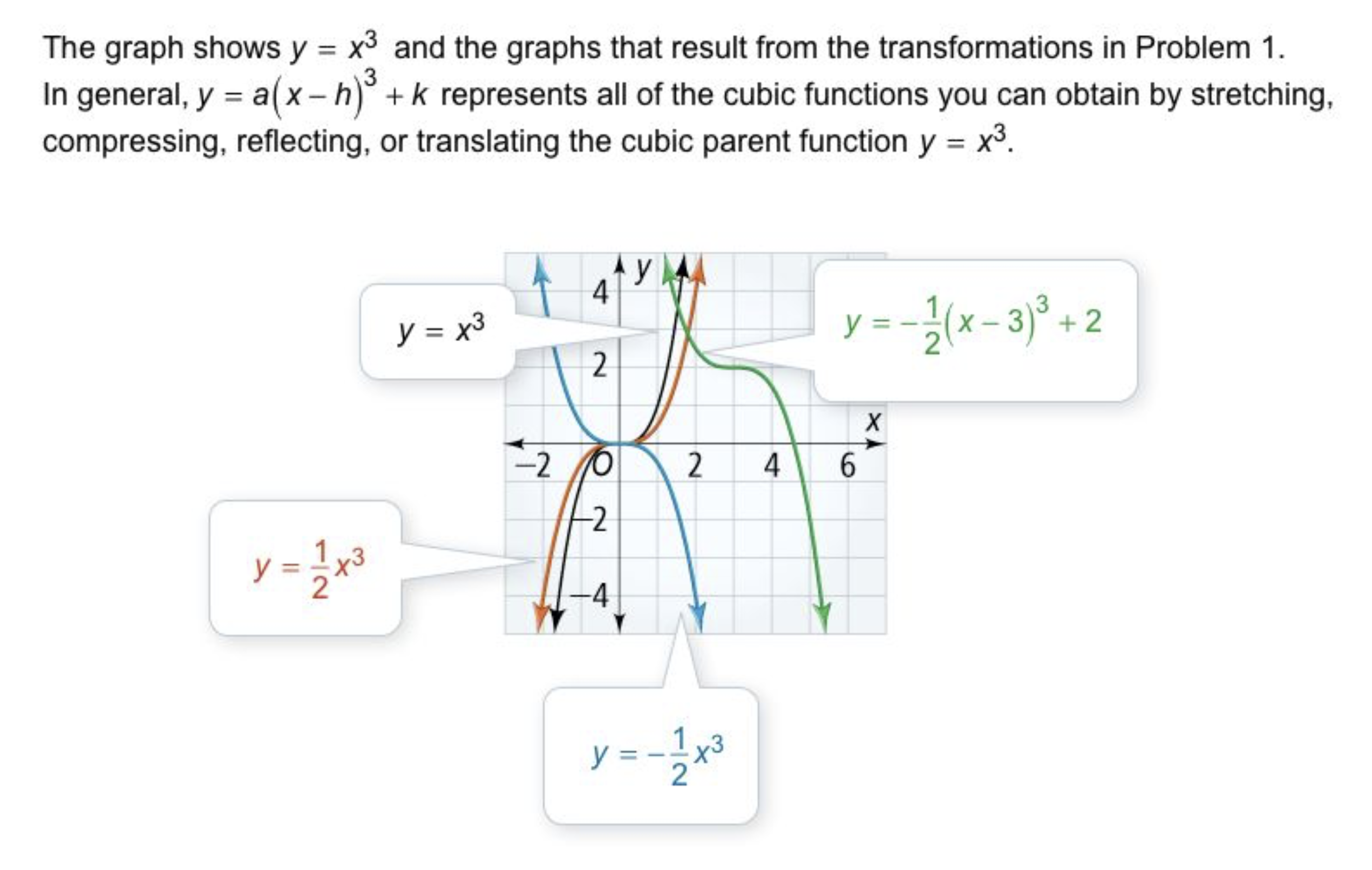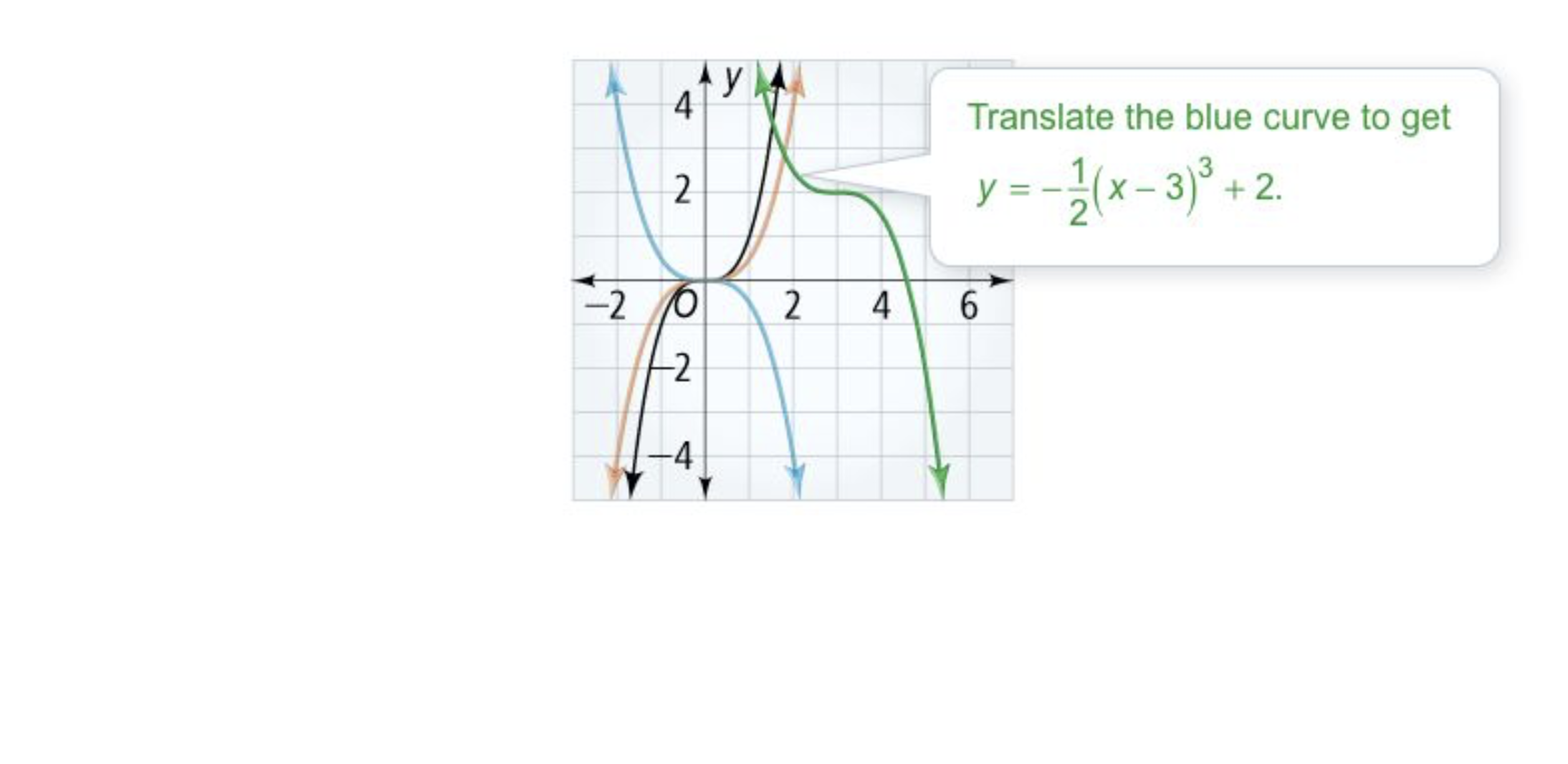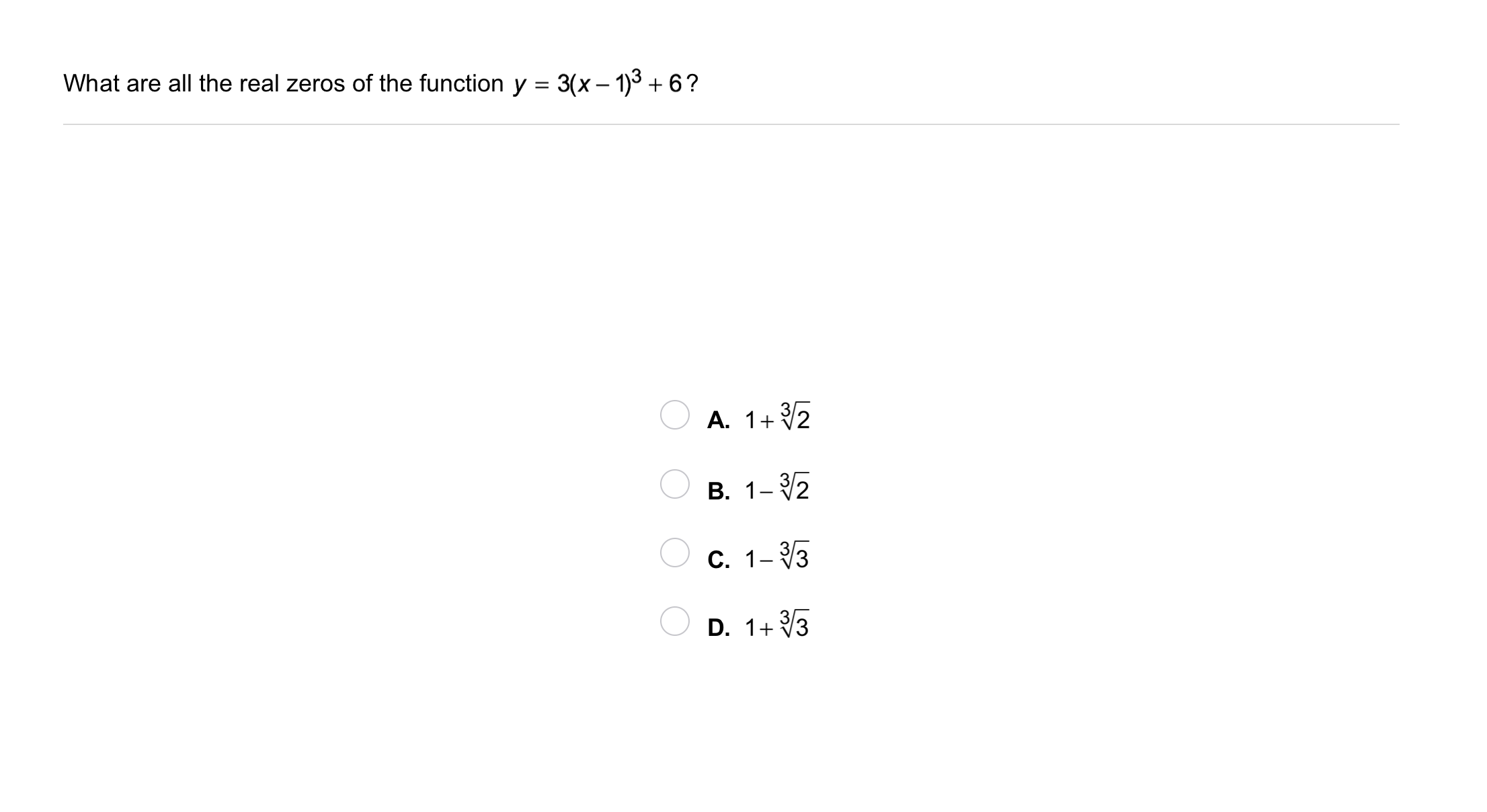3
3
10 pts
Problem 2 Got It?
A
B
C
D4
10 pts
Problem 3 Got It? What is a quartic function f(x) with only two real zeros, x = 0 and x = 6?

5
10 pts
Problem 3 Got It? Reasoning: Does -f(x) have the same zeros? Explain.
No; -f(x) is f(x) reflected across the y-axis, so the zeros will not stay the same.
Yes; -f(x) is f(x) reflected across the x-axis, so the zeros will stay the same.
Yes; -f(x) is f(x) reflected across the y-axis, so the zeros will stay the same.
No; -f(x) is f(x) reflected across the x-axis, so the zeros will not stay the same.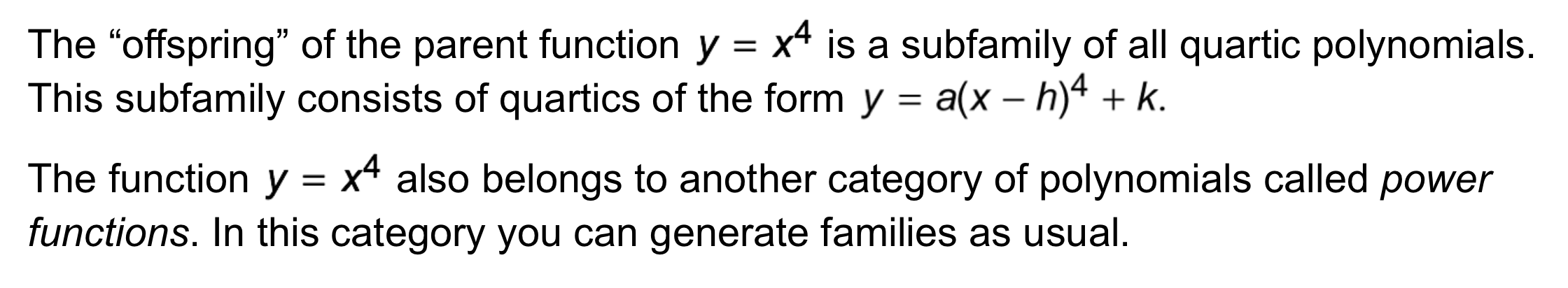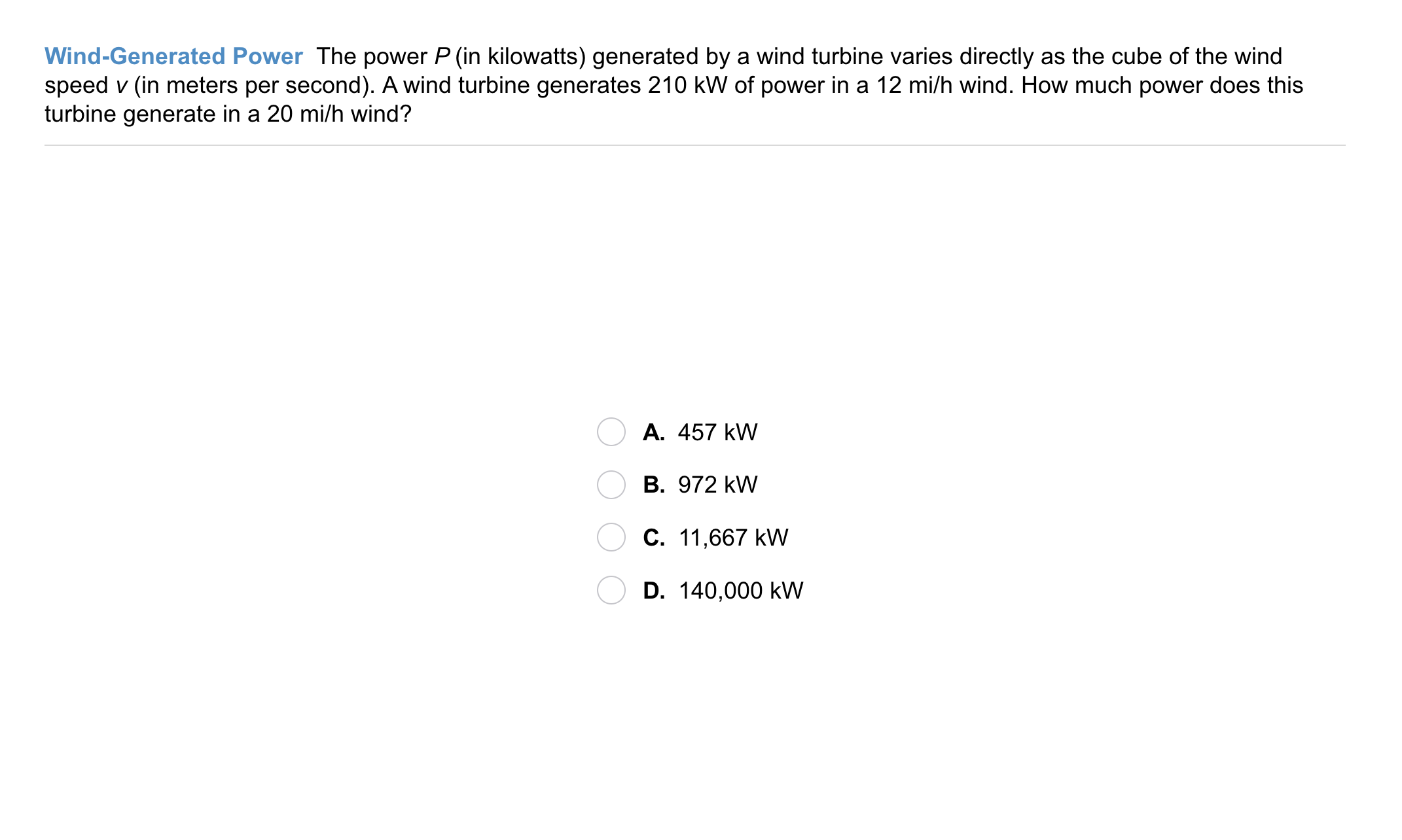6
6
10 pts
Problem 4 Got It?
A
B
C
D7
7
10 pts
A
B
C
D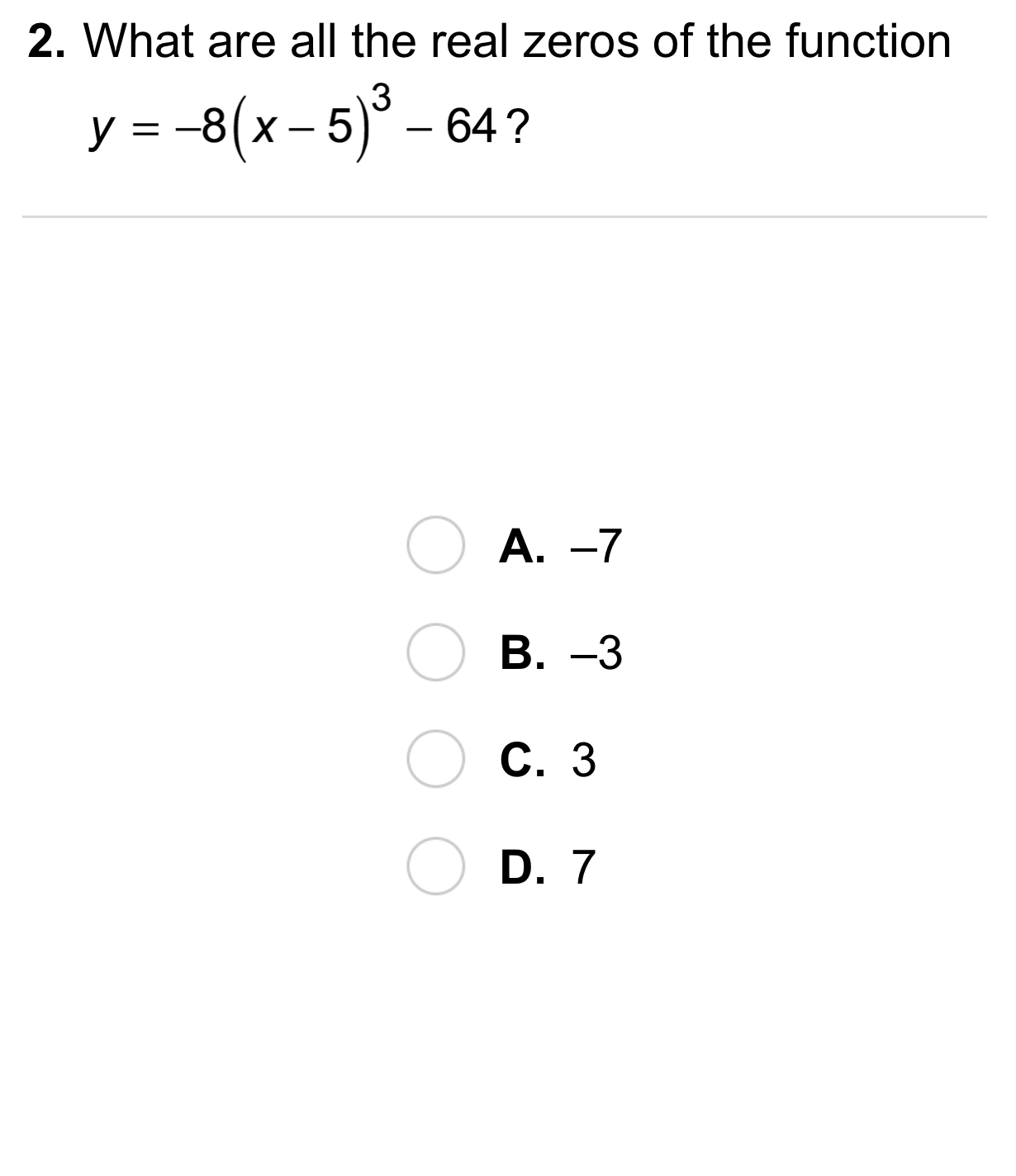8
8
10 pts
A
B
C
D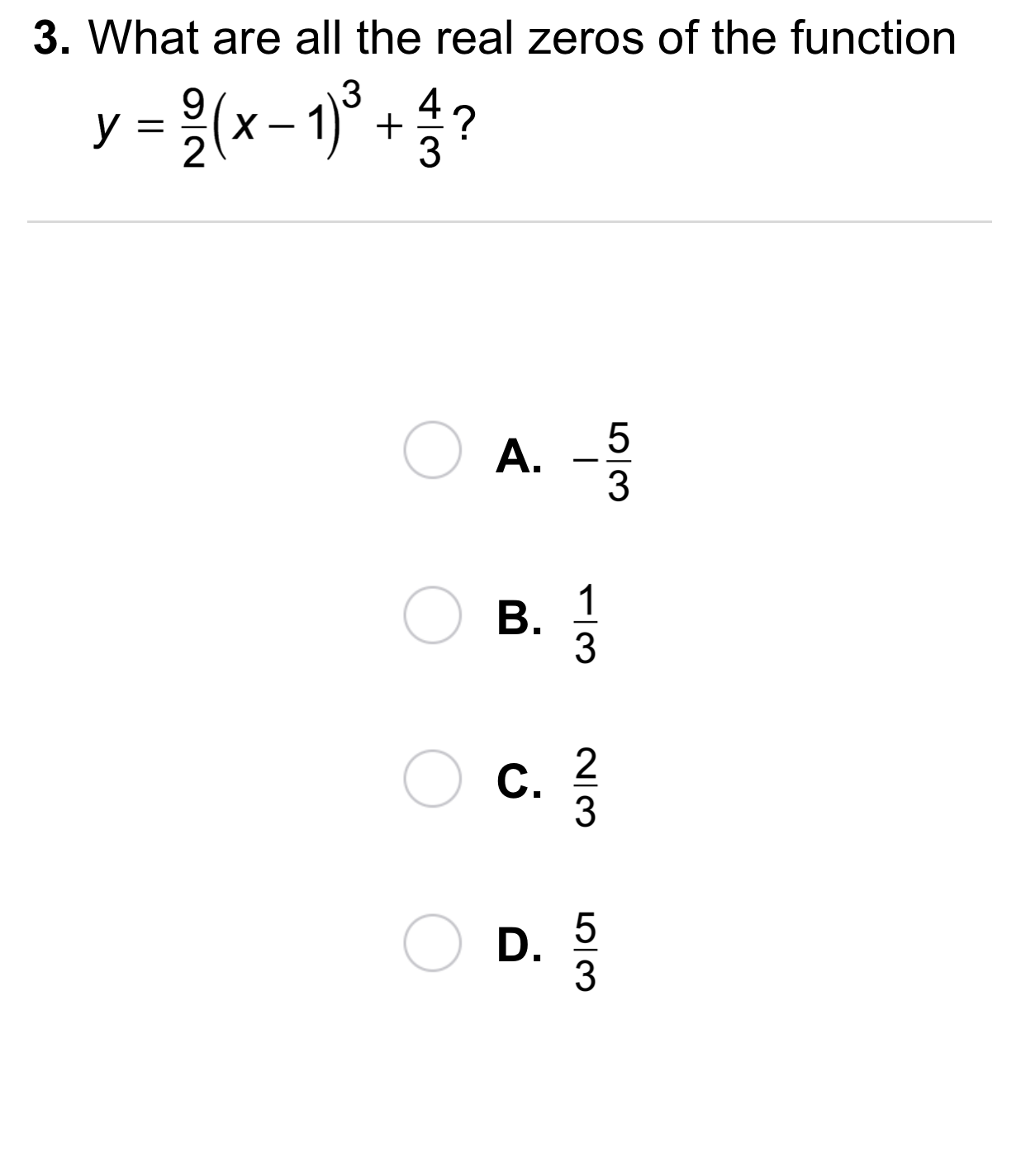9
9
10 pts
A
B
C
D10
5 pts
Vocabulary: Is the function below an example of a power function?

Yes
No
11
10 pts
12
10 pts
Error Analysis: Your friend says that he has found a way to transform the graph of the function below to obtain three real roots. Using the graph of the function, explain why this is impossible.
You may use the embedded Desmos utility below to graph the function.

The outlined content above was added from outside of Formative.
13
10 pts
Compare and Contrast: How are the graphs of the two functions below alike? different? What transformation was used to get the second equation?
You may use the embedded Desmos utility above to graph the function.14
10 pts
Review Lesson 5-8: Create a table and plot the points on the coordinate plane. Find and graph a polynomial function that passes through all of the given points. Zoom and pan your graph to establish an appropriate viewing window.

15
10 pts
Review Lesson 5-8: Create a table and plot the points on the coordinate plane. Find and graph a polynomial function that passes through all of the given points. Zoom and pan your graph to establish an appropriate viewing window.

16
10 pts
Review Lesson 2-4: Graph the lines described below on the same coordinate plane. Zoom and pan your graph to establish an appropriate viewing window.

17
10 pts
Review Lesson 2-1: Classify each relation.

• Function
• Not a function18
10 pts
Vocabulary Review: Identify the constant in the expression.

3

-3
2
1
19
10 pts
Vocabulary Review: Identify the expressions that do not contain a constant term. Select all that apply.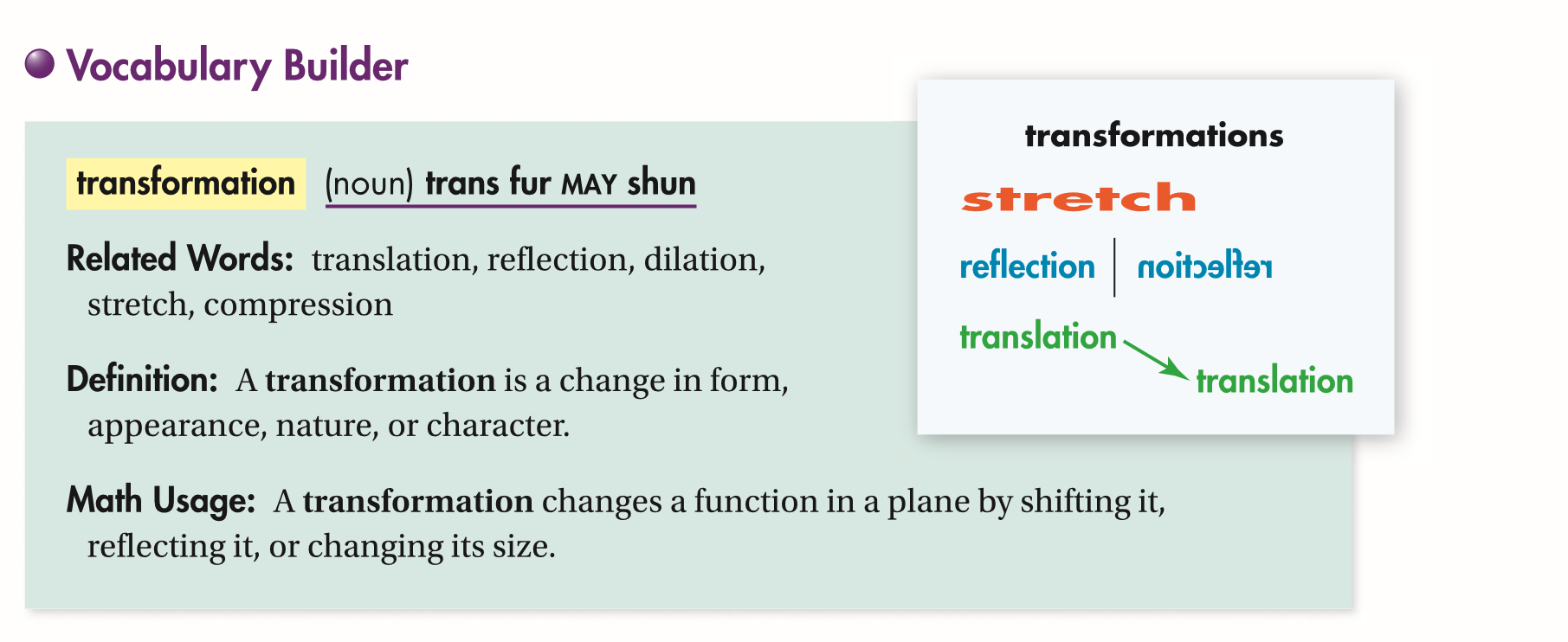20
10 pts
Use Your Vocabulary: Identify the translation, reflection, stretch, and compression of the word Algebra.

• Translation
• Reflection
• Stretch
• Compression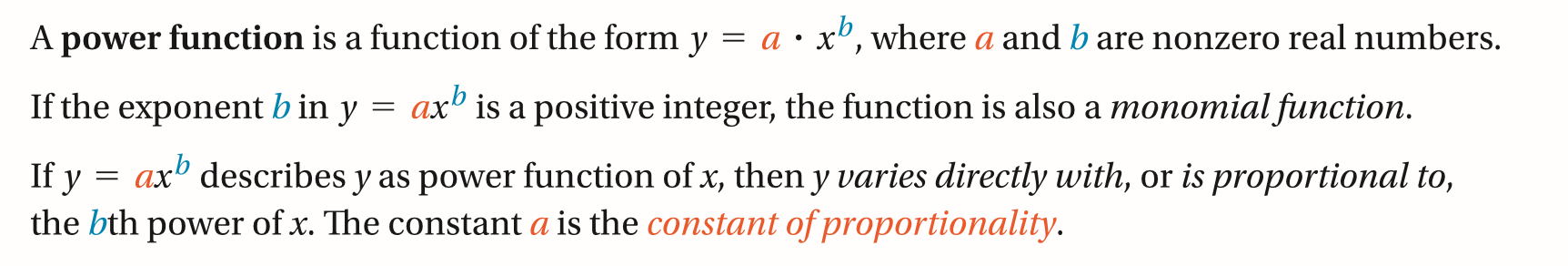21
21
10 pts
Key Concept: Identify the function(s) that are NOT Power Functions.

22
100 pts
Notes: Take a clear picture or screenshot of your Cornell notes for this lesson. Upload it to the canvas. Zoom and pan as needed.